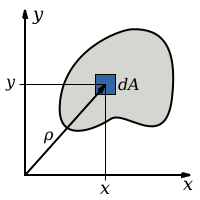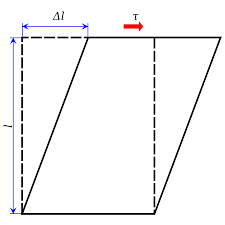## How to Calculate and Solve for Polar Moment of Inertia | Material SelectionThe image above represents polar moment of inertia.

To compute for polar moment of inertia, one essential parameter is needed and this parameter is Radius (r).

The formula for calculating polar moment of inertia:

J = πr4/2

Where:

J = Polar Moment of Inertia

Let’s solve an example;
Find the polar moment of inertia when the radius is 22.

This implies that;

J = πr4/2
J = π(22)4/2
J = π(234256)/2
J = 735936.928/2
J = 367968.46

Therefore, the polar moment of inertia is 367968.46 m4.

## How to Calculate and Solve for Shear Stress | Material SelectionThe image above represents shear stress.

To compute for shear stress, three essential parameters are needed and these parameters are Twisting Moment (M), Radius (r) and Polar Moment of Inertia (J).

The formula for calculating shear stress:

τ = Mr/J

Where:

τ = Shear Stress
M = Twisting Moment
J = Polar Moment of Inertia

Let’s solve an example;
Find the shear stress when the twisting moment is 12, the radius is 8 and the polar moment of inertia is 14.

This implies that;

M = Twisting Moment = 12
J = Polar Moment of Inertia = 14

τ = Mr/J
τ = (12)(8)/14
τ = 96/14
τ = 6.85

Therefore, the shear stress is 6.85 Pa.

Calculating the Twisting Moment when the Shear Stress, the Radius and the Polar Moment of Inertia is Given.

M = τJ / r

Where;

M = Twisting Moment
τ = Shear Stress
J = Polar Moment of Inertia

Let’s solve an example;
Find the twisting moment when the shear stress is 10, the radius is 6 and the polar moment of inertia is 4.

This implies that;

τ = Shear Stress = 10